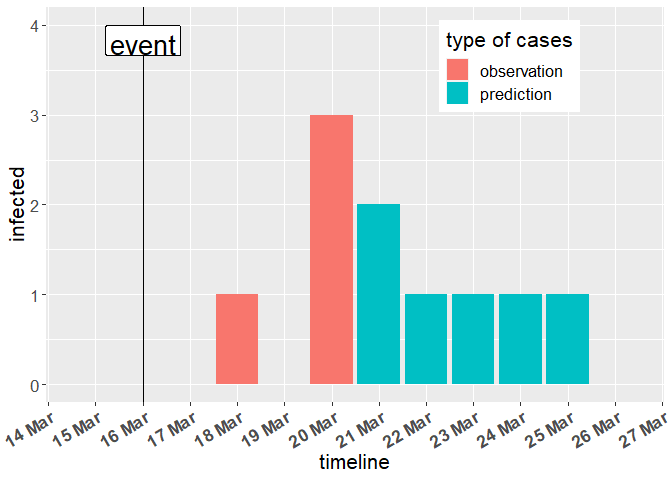# Statistical Modelling for Infectious Disease Management - Prediction of future infections in a group

## Question

A family party with 25 participants took place on the 15.03.2022 in a closed room and no masks were worn. In the following days some participants start to show symptoms of a COVID-19 infection: one on 18.03.2022 and three on 20.03.2022. How many further symptomatic infections can be expected in the following days?

## Calculating a prediction of the total number of infections with get_expected_total_infections

The function get_expected_total_infections() can be used to give a first answer to the question. It returns a prediction how many people in the group are expected to show symptoms in total.

### Inputs

The following input values are necessary for the function get_expected_total_infections() to work:

The group_size is the number of people participating in the event, including all observed infections.

The last_day_reported_infection is the number of days after the event when the last symptom begin was observed and the total_reported_infections is the total number of observed infections so far.

Finally, meanlog and sdlog are the mean and standard deviation parameters of the log-normal distribution for the incubation time derived from the paper Xin et al. .

### Methodology

Based on the incubation time distribution one can calculate the percentage of all symptomatic infections that will have their symptom onset up to the last_day_reported_infection. Then, said percentage is combined with the total_reported_infections to calculate the total symptomatic infections. The minimum between the result and the group_size is returned, because the group_sizeis obviously an upper bound for the total infections.

### Output

group_size <- 25
last_day_reported_infections <- 5 # day 0 = event day
total_reported_infections <- 4
meanlog <- 1.69
sdlog <- 0.55

predicted_total_infections <- get_expected_total_infections(group_size,
last_day_reported_infections,
total_reported_infections,
meanlog,
sdlog)
print(predicted_total_infections)
#>  10

The output represents how many people are expected to get a symptomatic infection. In the example 10 infections are predicted in total, which implies one can expect 6 further people starting to show symptoms in the next days because 4 infections were already observed.

## Generating a vector with number of people starting to show symptoms on each day using predict_future_infections

The function predict_future_infections() can be used to give a more detailed answer. It creates a vector containing the predicted number of further people starting to show symptoms on each of the days after the event.

### Inputs

Multiple arguments are necessary for the function predict_future_infections() to work:

The last_day_reported_infection is the number of days after the event when the last symptom begin was observed and the total_reported_infections is the total number of observed infections so far.

Then, the total_expected_infections is needed, which defines the total number of expected infections, including the ones already observed. One can use the output of get_expected_total_infections() or an own estimation based on e.g. reported symptomatic infection rates in a population of interest. If the output of get_expected_total_infections() is used, then it should be based on the same meanlog and sdlog as in the call to predict_future_infections().

Finally, meanlog and sdlog are the mean and standard deviation parameters of the log-normal distribution for the incubation time.

### Methodology

The function predict_future_infections() uses the function get_incubation_day_distribution() to get a vector of day-specific probabilities of symptom onset, given that a person will develop symptoms. Default values of the log-normal distribution for the incubation time used in that function are taken from the paper Xin et al. .

Starting on the first day after the last_day_reported_infection, the probability of symptom onset on a particular day, given that no symptoms occurred so far, is multiplied by the number of further expected infections and rounded upwards to receive the expected number of people starting to show symptoms on that day. The probability that no symptoms occurred so far can be calculated by 1 minus the sum of symptom onset probabilities for all previous days. The number of further expected infections is simply total_expected_infections minus total_reported_infections. The latter is afterwards raised by the predicted number of people with symptom onset on the day currently looked at, so that afterwards the next day can be treated.

When at some point the updated total_reported_infections is not smaller than total_expected_infections anymore, a 0 is inserted to signal that all further expected symptomatic infections are allocated and the loop is stopped.

### Output

last_day_reported_infections <- 5 # day 0 = event day
total_reported_infections <- 4
total_expected_infections <- get_expected_total_infections(25, 5, 4)
meanlog <- 1.69
sdlog <- 0.55

predicted_daily_infections <- predict_future_infections(last_day_reported_infections,
total_reported_infections,
total_expected_infections,
meanlog,
sdlog)
print(predicted_daily_infections)
#>   0 0 0 0 0 2 1 1 1 1 0

The function predict_future_infections() creates a vector with values representing the expected distribution of new symptomatic infections over the days after the event. Up to the last_day_reported_infections the entries are 0 because only infections in the future are predicted.

## An example for visualizing the output of predict_future_infections

Source
data <- data.frame("Erkrankungsdatum" = as.Date("2022-03-15") + 0:5,
"Neue_Faelle" = c(0, 0, 0, 1, 0, 3))
expected <- data.frame("Erkrankungsdatum" = as.Date("2022-03-15") + 1:(length(predicted_daily_infections)),
"ErwarteteWeitereFaelle" = predicted_daily_infections)
g <- ggplot(expected) +
geom_bar(
data = data,
aes(Erkrankungsdatum,
Neue_Faelle,
fill = "observation"),
stat = 'identity'
) +
geom_bar(
data = expected,
aes(Erkrankungsdatum,
ErwarteteWeitereFaelle,
fill = "prediction"
),
stat = 'identity'
) +
geom_vline(xintercept = expected$Erkrankungsdatum) + geom_label(aes(x = expected$Erkrankungsdatum, y = data\$Neue_Faelle + 4, label = "event"),
colour = "black", fill = "white", vjust = 1, size = 7) +
scale_y_continuous(breaks = function(x) unique(
floor(pretty(seq(0, (max(x) + 1) * 1.1))))) +
scale_x_date(date_breaks = "1 day", date_labels = "%d %b", minor_breaks = NULL) +
ylab("infected") +
xlab("timeline") +
labs(fill = 'type of cases') +
theme(legend.position = c(0.75,0.85), text = element_text(size = 16),
axis.text.x = element_text(face = "bold", angle = 30, hjust = 1))
return(g)## Literature

 Xin H, Wong JY, Murphy C, Yeung A, Ali ST, Wu P, Cowling BJ. The Incubation Period Distribution of Coronavirus Disease 2019: A Systematic Review and Meta-Analysis. Clinical Infectious Diseases. 2021; 73(12): 2344-2352.